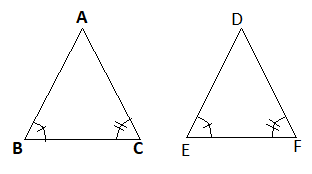### Common Errors in Secondary Mathematics

Common Errors Committed  by the  Students  in Secondary Mathematics   Errors  that students often make in doing secondary mathematics  during their practice and during the examinations  and their remedial measures are well explained here stp by step.  Some Common Errors in Mathematics

# CONGRUENCE OF TRIANGLES

Congruent figures, different congruence conditions, SSS, SAS, ASA, AAS and RHS congruence rules. Congruence of triangles class 9th and congruence of triangles for class 7th.

##CONGRUENCE OF TRIANGLES

## CONGRUENT FIGURES:-

Two  figures are said to be congruent if its all parts are equal. That is their all angles and all sides are equal.
Two squares of equal sides are congruent.
Two circles of equal radii are congruent.
Two equilateral triangles of equal sides are congruent.

## CONGRUENCE OF TRIANGLES :-

Two triangles are congruent if their all sides and all angles are equal.

## The main Congruence conditions are as follows:-

1) Side-Side-Side Congruence condition
2) Side-Angle-Side Congruence rule
3) Angle-Side-Angle Congruence rule
4) Angle-Angle-Side Congruence rule
5) Right angle-Hypotenuse-One side Congruence rule

# Explanation of each rule of congruency of triangles

## 1) Side-Side-Side Congruence condition(SSS ≅ Rule):-

If three sides of one triangle are  equal to the corresponding three sides of other triangle then triangles are said to be congruent and the condition is called SSS ≅ condition.In ABC and DEF
AB = DE
BC = EF
AC = DF
∴ by SSS ≅ rule ABC ≅  DEF

## 2) Side-Angle-Side Congruence rule(SAS ≅ Rule)

If two sides and included angle of one triangle are equal to the corresponding two sides and included angle of other triangle then triangles are said to be congruent and the condition is called SAS ≅ condition.In
ABC and DEF
AB = DE
AC = DF
∠A = ∠D
∴ by SAS ≅ rule ABC  DEF

## 3) Angle-Side-Angle Congruence rule:-

If two angles and included side of one triangle are  equal to the corresponding two angles and included side of other triangle then triangles are said to be congruent and the condition is called ASA ≅ condition.In ABC and DEF
∠B = ∠E
BC = EF
∠C = ∠F
∴ by ASA ≅ rule ABC  DEF

## 4) Angle-Angle-Side Congruence rule:-

If two angles and one side of one triangle are  equal to the corresponding two angles and one side of other triangle then triangles are said to be congruent and the condition is called    AAS≅ condition.In ABC and DEF
∠A = ∠D
∠B = ∠E
BC = EF

by AAS ≅ rule ABC  DEF

## 5) Right angle-Hypotenuse-One side Congruence rule:-

In Right angled triangles if hypotenuse and one side of one triangle are respectively equal to the corresponding hypotenuse and one side of other triangle then triangles are congruent and the condition is called RHS ≅ condition.
In ABC and DEF
∠C = ∠F.....(each = right angle
AB = DE
AC = DF
by RHS ≅ rule ABC  DEF

## Properties of triangle

Property 1

Here In △ ABC,  ∠ACD is an exterior angle and ∠A and ∠B are interior opposite angles. So
∠ACD = ∠A + ∠B

Property 3
Angle opposite to equal sides of triangle are equal and sides opposite to equal angles of triangle are equal.

Property 4
In triangle angle opposite to larger side is large and smaller side is small.
In △ ABC, AC > AB  ⇒ ∠B > ∠C
AC > BC ⇒  ∠B > ∠A

Property 5
Sum of any two sides of a triangle is always greater than the third side.
AB + BC > AC
AB + AC > BC
AC + BC > AB

Property 6
If two sides of a triangle are given the range of third side is calculated as follows.
Difference of two given sides < Third side < Sum of two given sides
For example: If two sides of triangle are 5cm and 8cm the range of third side is given by
(8 - 5) cm < Third Side < (8 + 5) cm
3 cm < Third Side < 13 cm
Third side lie between 3cm and 13 cm

🙏